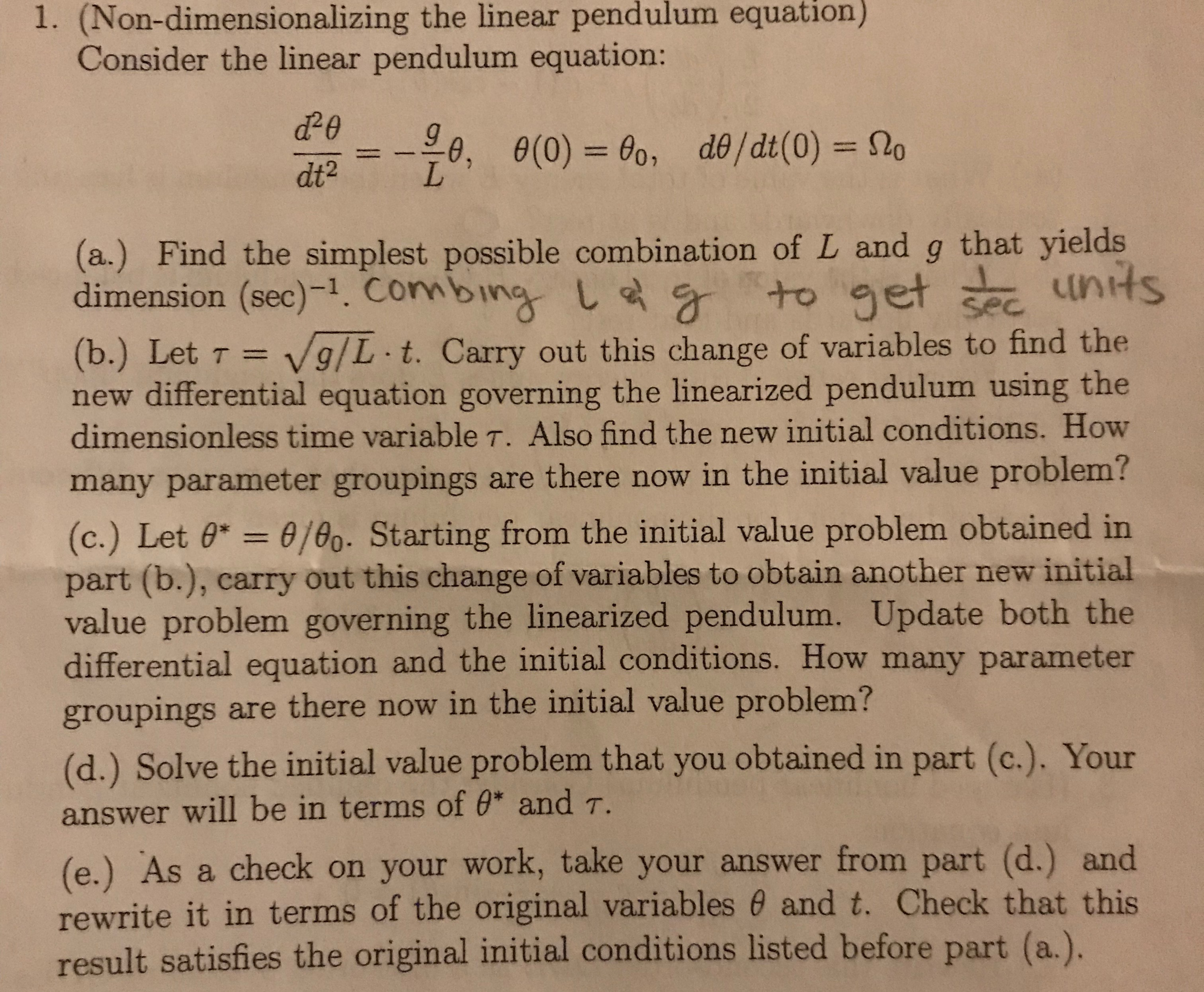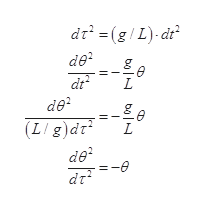# 1. (Non-dimensionalizing the linear pendulum equationConsider the linear pendulum equation:e(0)= 00, de /dt (0) = 2oLdt2(a.) Find the simplest possible combination of L and g that yieldsdimension (sec)-1. Connbing L to getunitsSec(b.) Let Tnew differential equation governing the linearized pendulum using thedimensionless time variable T. Also find the new initial conditions. HowV9/L t. Carry out this change of variables to find themany parameter groupings are there now in the initial value problem?(c.) Let 0* = 0/00. Starting from the initial value problem obtained inpart (b.), carry out this change of variables to obtain another new initialvalue problem governing the linearized pendulum. Update both thedifferential equation and the initial conditions. How many parametergroupings are there now in the initial value problem?(d.) Solve the initial value problem that you obtained in part (c.). Youranswer will be in terms of 0* and T.(e.) As a check on your work, take your answer from part (d.) andrewrite it in terms of the original variables 0 and t. Check that thisresult satisfies the original initial conditions listed before part (a.).

Question
13 views

pleas ehelp me answer these questions.

a,b,c,d,and e.

thank you!help_outlineImage Transcriptionclose1. (Non-dimensionalizing the linear pendulum equation Consider the linear pendulum equation: e(0)= 00, de /dt (0) = 2o L dt2 (a.) Find the simplest possible combination of L and g that yields dimension (sec)-1. Connbing L to get units Sec (b.) Let T new differential equation governing the linearized pendulum using the dimensionless time variable T. Also find the new initial conditions. How V9/L t. Carry out this change of variables to find the many parameter groupings are there now in the initial value problem? (c.) Let 0* = 0/00. Starting from the initial value problem obtained in part (b.), carry out this change of variables to obtain another new initial value problem governing the linearized pendulum. Update both the differential equation and the initial conditions. How many parameter groupings are there now in the initial value problem? (d.) Solve the initial value problem that you obtained in part (c.). Your answer will be in terms of 0* and T. (e.) As a check on your work, take your answer from part (d.) and rewrite it in terms of the original variables 0 and t. Check that this result satisfies the original initial conditions listed before part (a.). fullscreen
check_circle

Step 1

(a) Write the dimension of L and g

Thus,

The simplest combination of L and g that yields units of (sec)-1 is (g/L)-1/2.

Step 2

(b) Use substitution of variable in the given equationhelp_outlineImage Transcriptionclosedr (g/ L) d de2 dt de2 (L/g)dr L L de =-e dr D | fullscreen
Step 3

The new boundary conditions for ...

### Want to see the full answer?

See Solution

#### Want to see this answer and more?

Solutions are written by subject experts who are available 24/7. Questions are typically answered within 1 hour.*

See Solution
*Response times may vary by subject and question.
Tagged in

### Science